# Example 4: Moment vs. Curvature Curve for specific reinforcement ratios

Watch the video of this example >

###### Data for Cross Section Analysis & Design application

Aforementioned Example 3 will be extended here, in order to have Moment vs. Curvature diagrams calculated for different reinforcement ratios. The used cross section is similar to that defined in Example 3.

###### Moment vs. Curvature diagrams data

The Moment vs. Curvature diagram for bending about Y axis is requested for the following reinforcement ratio values:

• 0.5%
• 1.0%
• 1.5%

#### Opening a file from disk

First of all we click on the File menu and select Open in order to open the file we created in Example 3.

#### Unlock the model

Afterwards, if the model is locked, we click on Edit -> Unlock model, in order to modify the geometry of the cross section.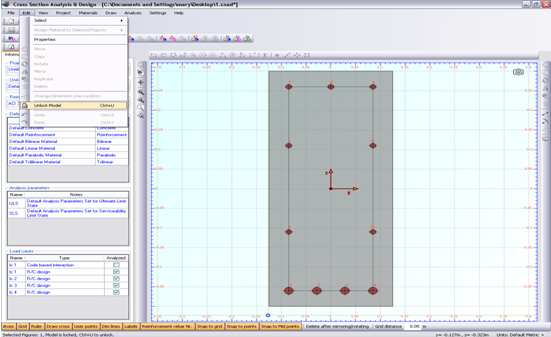In the popup window, we choose Yes to unlock the model.#### Definition of a new load case

We click on the Analysis -> Moment Curvature -> Load cases menu item and next on the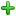button in order to insert a new load case “lc 2”. Load case “lc 2” contains information regarding the reinforcement ratio levels used for the analysis. We change the Analysis Parameters to “ULS” and keep the remaining values. Afterwards, the different reinforcement ratio levels can be defined by clicking on the “Options” button of the “lc 2” row.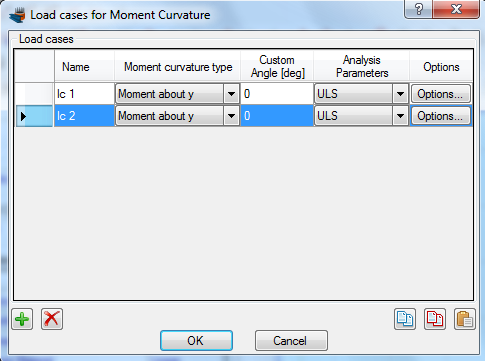On the form following that shows, we choose the “Alternate Reinforcement Ratio” option and provide the reinforcement ratio values on the list on the right.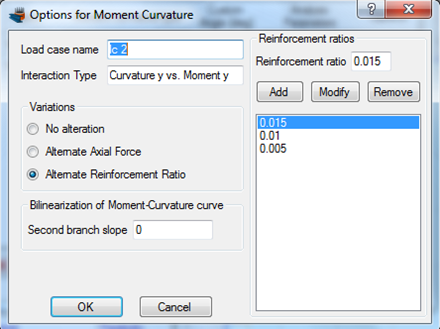We click OK to close the form.

#### Carry out the analysis

We just click Analysis -> Moment Curvature -> Analyze, to perform the Moment Curvature analysis.

#### Results

The results can be obtained from the Analysis -> Moment Curvature -> Show results menu item. On this form, we choose “lc 2” in the Load case list at its top left corner in order to view the results of the load case defined previously.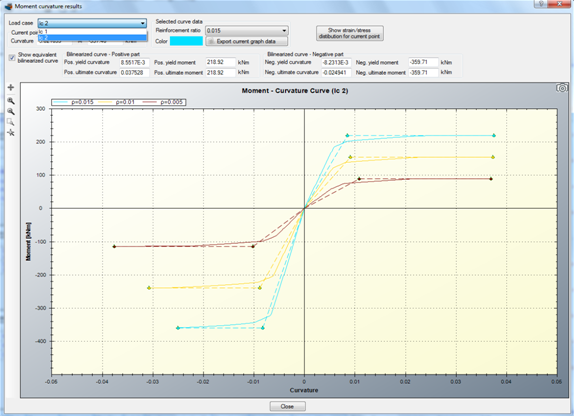The Moments vs. Curvature diagrams for the three specified reinforcement ratio levels are shown in the form. We note that the equivalent bilinearized curves (same color, but dashed) have also been calculated. These can disappear by unchecking the “Show equivalent bilinearized curve” box.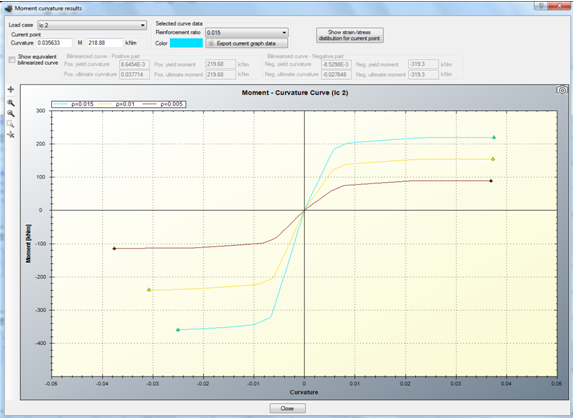The color of each curve can be controlled from the corresponding “Color” box after selecting the curve to modify by its reinforcement ratio level.

Note: All reinforcement size constraints are respected when a reinforcement ratio is calculated. Thus, the side reinforcement bars are constant and bottom reinforcement area is 50% greater of the top ones (as they grow factor has been specified equal to 1.5).

#### Section stress distribution for a specified point on Moments vs. Curvature curve

Assuming we would like to display the strain distribution and stress contour for a specific point on the diagram for the curve which refers to reinforcement ratio level of 0.015.

First of all we select this value from the related list as shown in the picture below.Afterwards we move the mouse over the the graph.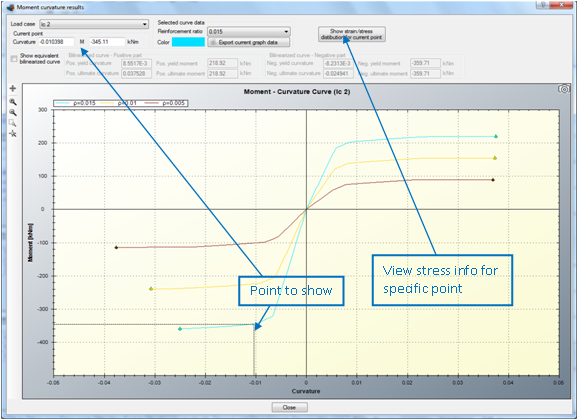By clicking of “Show strain/stress distribution for current point”, a form is shown, which represents the following data:

• Neutral axis location
• Strain distribution
• Equivalent force couple
• Stress contour on the cross section area
• Reinforcement forces/stresses
• Stress information per material

The form is shown below.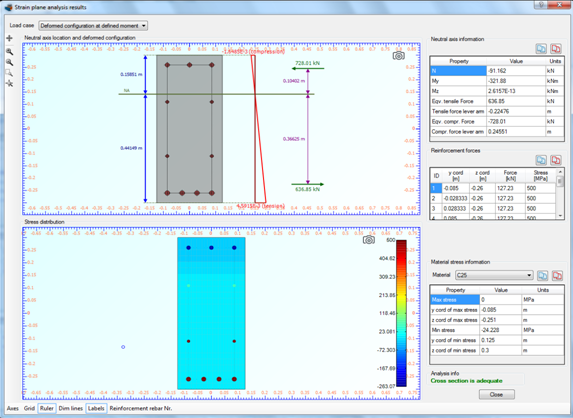## Watch the video of this exampleHave any questions? Do not hesitate to contact us! Contact  us ›